# Sieve of Eratosthenes

Sieve of Eratosthenes is a wonderful technique to get a list of prime numbers in the range (1, n]. It also supports testing for primality of any number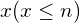in O(1) after pre-processing. The pre-processing phase has time complexity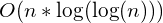in original version and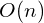in enhanced version.

Original Sieve of Eratosthenes

This technique relies on one simple observation: if x is a composite number (or we can say: if x is not a prime number), then there exists a prime number p which is a divisor of x, and of course, p < x.

So, if for every prime number p such that p < x, we mark all numbers that p divides as composite. Then we know that x is a composite number if and only if x is marked, right?

struct PrimeSieve {

int n;
bool *mark;
vector<int> primes;

PrimeSieve() {}

void precompute(int n_) {

// initialize variables
n = n_;
mark = new bool[n+1];
fill(mark, mark + n + 1, true);
primes.clear();

// find prime numbers and mark composite numbers
for (int x = 2; x <= n; ++x) {

if (mark[x]) {

primes.push_back(x);

for (int y = x*2; y <= n; y += x)
mark[y] = false;
}

}

}

vector<int> getPrimeList() {
return primes;
}

bool isPrime(int x) {

if (x < 2)
return false;

assert(x <= n);

return mark[x];

}

};

Enhanced Sieve of Eratosthenes

One more observation will help us further improve our sieve!

In the original sieve, each composite number is marked by all of its prime factors.
For example, a composite number u = 1638. We have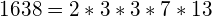, so u has 4 prime factors: 2, 3, 7 and 13.
2 is prime, hence it marks all the number it divides as composite. And because 2 divides 1638, it marks 1638 as a composite number.
3 is prime, hence it marks all the number it divides as composite. And because 3 divides 1638, it marks 1638 as a composite number.
7 is prime, hence it marks all the number it divides as composite. And because 7 divides 1638, it marks 1638 as a composite number.
13 is prime, hence it marks all the number it divides as composite. And because 13 divides 1638, it marks 1638 as a composite number.

Have you seen it? We are making redundant efforts. We mark 1638 as composite a total of 4 times. Now the observation: We can mark 1638 only 1 time, we can have just the smallest prime that divides 1638 (which is the number 2) marks it as a composite number. We do that for all numbers, and hence reduce the complexity of our sieve to just.

How do we do that? A composite number, let’s call it u. We know that, since u is composite, it should be divided by at least 1 prime number. Let’s call lp as the smallest prime number that divides u. Say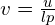.
We get the least prime number that divides v is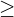lp because if it is < lp, then lp is not the smallest prime that divides u, hence contradiction.

So the algorithm comes in: For each prime number p, we mark as composite all number u such that p divides u and the least prime number that divides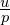isp. Or if we take another viewpoint: Let’s call the least prime that divides a number v is least_prime[p]. For each number v (we don’t care if v is composite or prime), we iterate through all prime numbers p such that p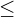least_prime[v] and mark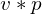as composite.

// Improved version of Sieve
// Support finding factorization of all numbers from 1 to n
// Usage: PrimeSieve2 sieve; sieve.precompute(n); sieve.isPrime(x); sieve.getFac(x);
struct PrimeSieve2
{
int n;
int *least_prime;
vector<int> primes;

PrimeSieve2() {}

void precompute(int n_) {
n = n_;
least_prime = new int[n+1];
fill(least_prime, least_prime + n + 1, 0);

for (int i = 2; i <= n; ++i) {

if (least_prime[i] == 0) {
least_prime[i] = i;
primes.push_back(i);
}

for (int j = 0;
j < primes.size() && primes[j] <= least_prime[i] && primes[j] * i <= n;
++j)
least_prime[i * primes[j]] = primes[j];
}
}

bool isPrime(int &x) {
if (x < 2)
return 0;
return least_prime[x] == x;
}

vector< pair<int, int> > getFac(int x) {
vector<pair<int, int> > fac;

if (x < 2) return fac;

while(x > 1) {
int d = least_prime[x];
int cnt = 0;
while(x % d == 0) {
x /= d;
cnt++;
}

fac.push_back({d, cnt});
}

return fac;
}

};